# Test: Inverters

## 10 Questions MCQ Test Topicwise Question Bank for Electronics Engineering | Test: Inverters

Description
Attempt Test: Inverters | 10 questions in 30 minutes | Mock test for Electrical Engineering (EE) preparation | Free important questions MCQ to study Topicwise Question Bank for Electronics Engineering for Electrical Engineering (EE) Exam | Download free PDF with solutions
QUESTION: 1

### Assertion (A): For high power applications, inverters are used instead of transistors. Reason (R): For high power applications, inverter is operated in active region.

Solution:

In low-power electronic circuits oscillators are used for converting dc power into ac power. These oscillator use transistors for converting dc voltage into sinusoidal ac voltage. Since transistor is used in active region, therefore there is substantial loss of power which decreases efficiency. In high power applications inverters are used instead of transistors and the inverters operate in saturation region or cut-off region. Thus, assertion is true but reason is false.

QUESTION: 2

Solution:
QUESTION: 3

### Consider the following statements associated with CSI and VSI: 1. In CSI, commutation is load dependent process. 2. Thyristorised current source fed inverter has inherent four quadrant operation. 3. In VSl dynamic braking is applicable during ac line failure. 4. MOSFET and transistor are more suitable for CSl compared to VSI. Which of the statements given above is/are correct?

Solution:

• In CSI at light load, commutation time is considerably increased, which can restrict the highest frequency. Hence, commutation is load dependent process in CSI. Thus, statement-1 is correct.
• A VSl requires an additional line commutated converter for reverse power flow while it is hot so for a CSI i.e. a CSI has inherent four quadrant operation. Thus, statement-2 is correct.
• In VSl, dynamic braking is applicable during ac line failure. Thus, statement-3 is correct.
• MOSFET and transistor are more suitable for VSl not for CSI because in CSI large transient voltage is produced during commutation. Hence, statement-4 is not correct.

QUESTION: 4

A single-phase half bridge inverter has supply voltage of 200 V. For a load resistance of 10 Ω, the output power is equal to

Solution:

Output is a square wave with an amplitude of 100V.
RMS value of a square wave is equal to its peak value.
So, rms value of output voltage is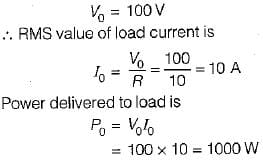QUESTION: 5

Assertion (A): The terminal voltage of a voltage source inverter remains substantially constant with variations in load.
Reason (R): Any short-circuit across the terminals of a voltage source inverter causes current to rise very fast.

Solution:

A voltage source inverter is one in which the d.c. source has small or negligible impedance. Due to low internal impedance, the terminal voltage of a VSI remains substantially constant with variations in load. Reason is also a correct -statement because due to low time constant of internal impedance any short-circuit across the terminals of a VSI causes current to rise very fast. Hence, both assertion and reason are true but reason is not the correct explanation of assertion.

QUESTION: 6

The single-phase half-bridge inverter has a resistive load of 10Ω and the centre-tap dc input voltage is 96 V. The fundamental power consumed by the load is

Solution:

The nth harmonic-component of output voltage is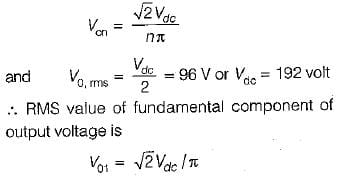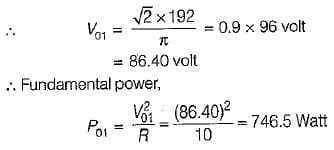QUESTION: 7

A single-phase half-bridge inverter has a resistive load of R = 3 Ω and the dc input voltage Vdc = 24 volts. The harmonic factor of the lowest order harmonic would be equal to

Solution:

The lowest harmonic is third harmonic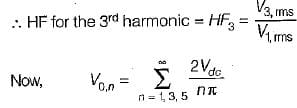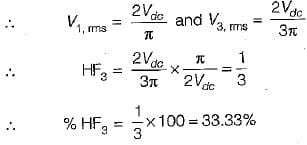QUESTION: 8

A single-phase transistorized bridge inverter has a resistive load of R = 3 Ω and the dc input voltage of 48 volts. The total harmonic distortion is approximately equal to

Solution: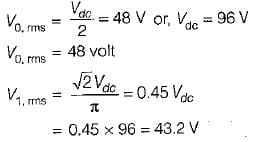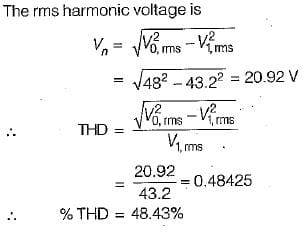QUESTION: 9

A full-bridge bipolar PWM inverter is fed from a 240 V battery and is driving an RL load. The fundamental output voltage for a modulation index of 0.8 is

Solution:

The fundamental output voltage for a modulation index of M is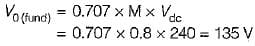QUESTION: 10

A current source inverter can be

Solution:

A CSl can be either load commutated or force commutated.Use Code STAYHOME200 and get INR 200 additional OFF Use Coupon Code